Concepts

Class 10
Chapter 1 Class 10 - Chemical Reactions and Equations

A chemical equation is the representation of a chemical reaction .

In the form of symbols and formulae

## Step 1

First we write chemical equation in word

Example

Let us consider the following chemical reaction;

Magnesium burns with Oxygen gas to form white colored powder called Magnesium Oxide

Writing in words;

Magnesium + Oxygen → Magnesium Oxide

Magnesium + Oxygen → Magnesium Oxide

Note (for the graphic designer) : Make an image of this word equation

While writing a chemical equation, it should be noted that;

1. Reactants are written on the left (LHS)
2. Products are written on the right (RHS)
3. There is a '+' sign between the reactants or products if there is more than one reactant/product.
4. There is an arrow '→' between the reactants and products which shows the direction of the reaction
5. If there is any additional force provided to the reaction , like heat or pressure, then it is to be written above or below the arrow .

Now writing a word equation can be tedious, and we can make a word equation shorter by using the unique symbols of the elements

## Step 2

We write symbols instead of words

Symbol for Magnesium is Mg

Symbol for Oxygen gas is O 2

## Formula for Magnesium Oxide

First we check the valency,

Valency of Magnesium is 2

Valency of Oxygen is 2

Formula for Magnesium Oxide is MgO as shown below

Writing Equation by using chemical formula

Magnesium + Oxygen → Magnesium Oxide

Mg + O 2         →          MgO

Mg + O 2         →          MgO

Note (for the graphic designer) : Make an image of this equation

This is our required chemical equation.

Step 3

Check and balance the equation

 Element Name No of Atoms in Reactant No of Atoms in Product Magnesium 1 1 Oxygen 2 1

Starting with Oxygen,

No. of atoms of Oxygen is not balanced

2 in the reactants side and 1 in the product side

So we multiply product by 2

Mg + O 2 2 MgO

Mg + O 2 2 MgO

We again check number of elements

 Element Name No of Atoms in Reactant No of Atoms in Product Magnesium 1 2 Oxygen 2 2

Now,

No. of atoms of Magnesium is not balanced

1 in the reactants side and 2 in product side

We try to make both same by multiplying reactant by 2

2 Mg + O 2 → 2MgO

2 Mg + O 2 2 MgO

Note (for the graphic designer) : Make an image of this equation

We again check number of elements

 Element Name No of Atoms in Reactant No of Atoms in Product Magnesium 2 2 Oxygen 2 2

## Step 4

Writing symbols of different states;

Following symbols are normally used

 State Symbol Solid s Liquid l Gas g Aqueous aq

Explanation

In our case

Magnesium burns with Oxygen to form white colored powder called Magnesium Oxide

Magnesium is solid, Oxygen is gas and Magnesium Oxide is also solid

Hence we write equation as

2Mg (s) + O 2 (g) → 2MgO (s)

2 Mg (s) + O 2 (g) → 2 MgO (s)

Note (for the graphic designer) : Make an image of this equation

Note: Aqueous state (aq) means presence of a substance as a solution in water

Another examples:

Green Plant make food by photosynthesis

following equation takes place

Carbon Dioxide + Water → Glucose + Oxygen

6CO 2 (g) + 6H 2 O (l) → C 6 H 12 O 6 (aq) + 6O 2 (g)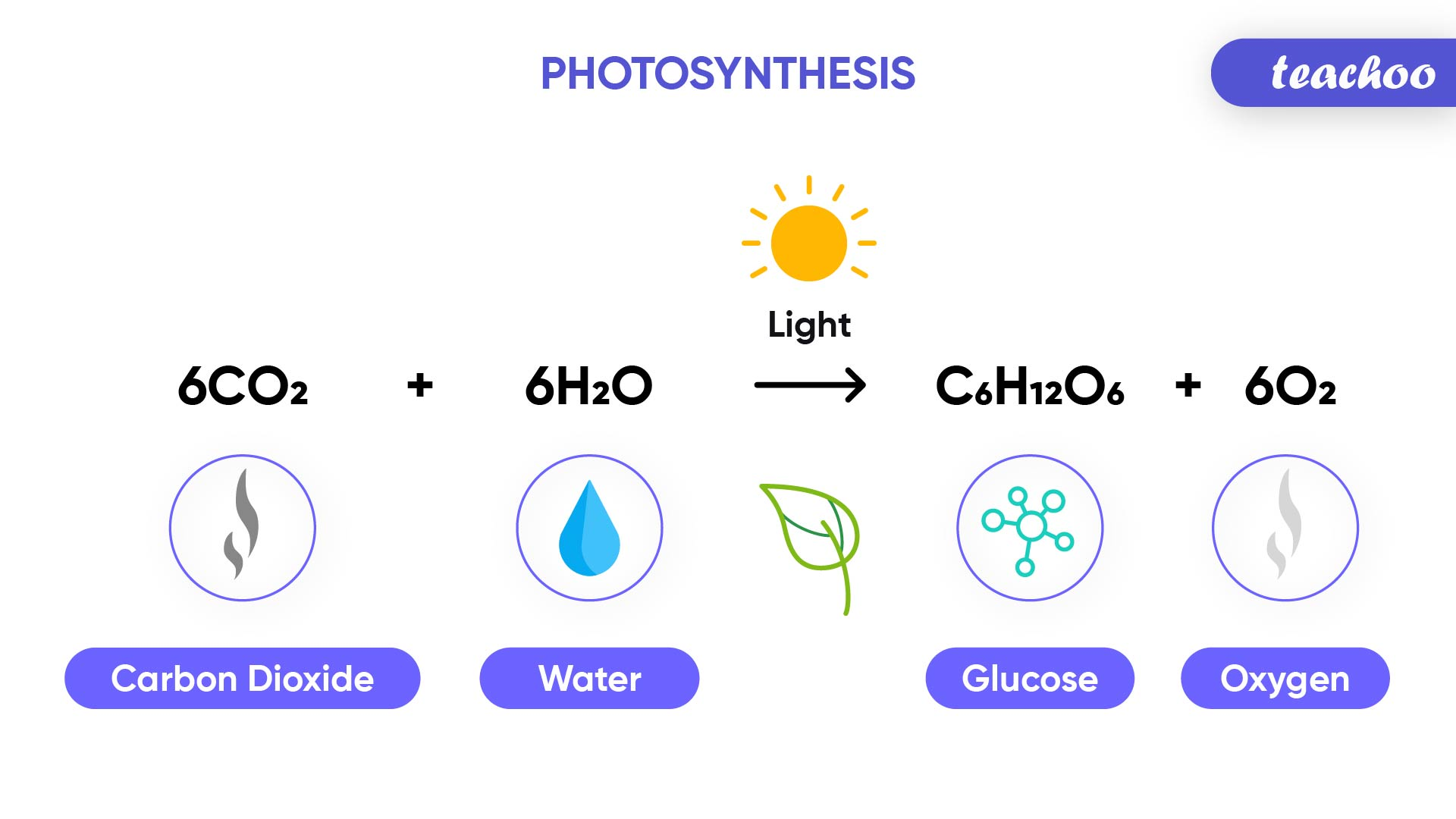This glucose is aqueous solution (glucose is present in water)

## Step 5

Sometimes extra information is given in the form of temperature, pressure, catalyst etc.

Catalyst : A catalyst is a substance that increases the rate of a chemical reaction without itself undergoing any permanent chemical change.

For example

Potassium Chlorate is heated in the presence of Magnesium Dioxide catalyst, it decomposes to produce Potassium Chloride and Oxygen gas

MnO 2

2KClO 3         →       2KCl + 3O 2

△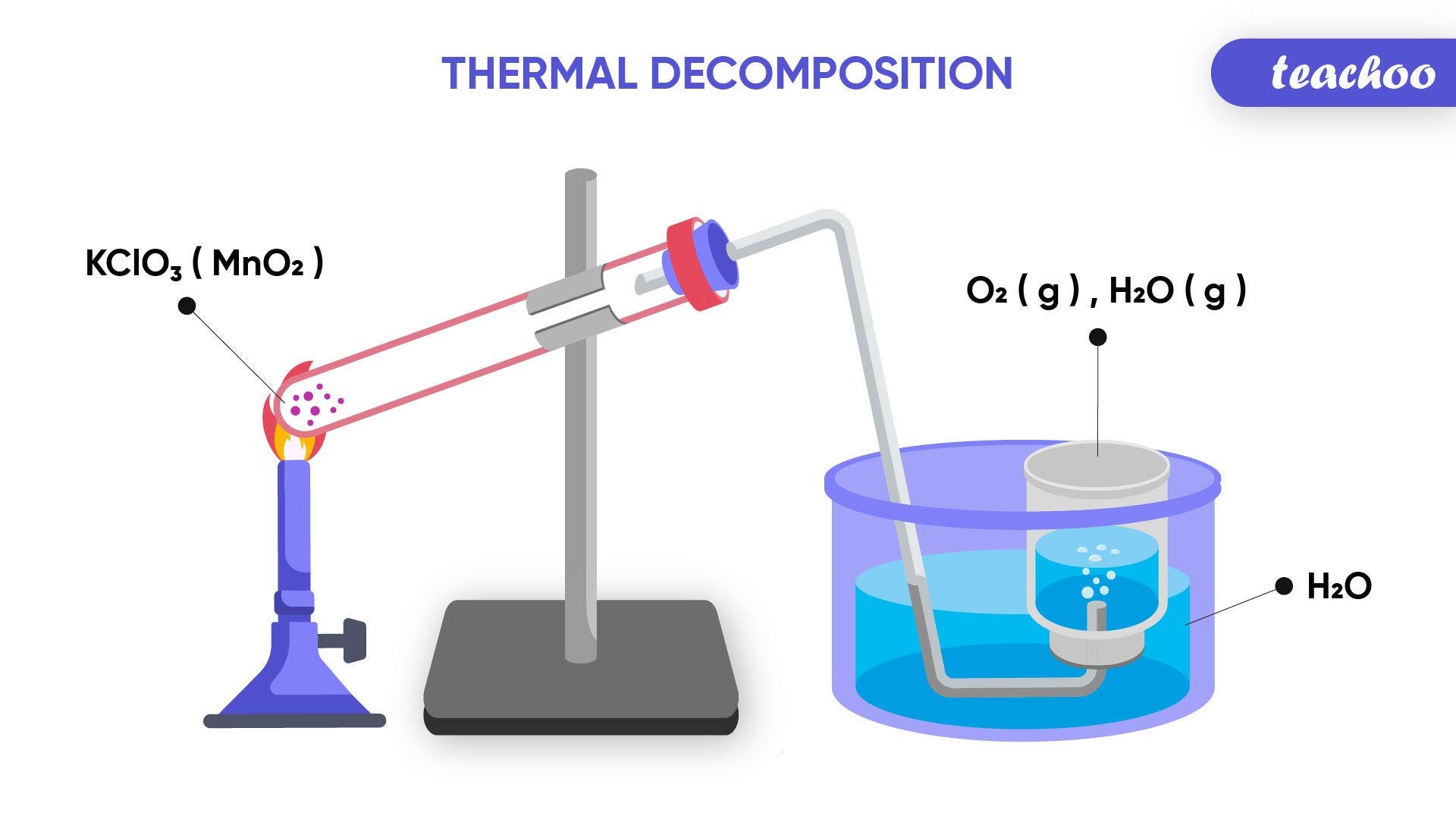Temperature -

Endothermic Reaction : Reaction in which heat energy is used is called Endothermic Reaction.

For example

When Calcium Carbonate is heated, it decomposes into Calcium Oxide and Carbon Dioxide

CaCO 3 (s) → CaO(s) + CO 2 (g)

Temperature -

Endothermic Reaction : Reaction in which heat energy is used is called Endothermic Reaction.

For example

When Calcium Carbonate is heated, it decomposes into Calcium Oxide and Carbon Dioxide

CaCO 3 (s) → CaO(s) + CO 2 (g)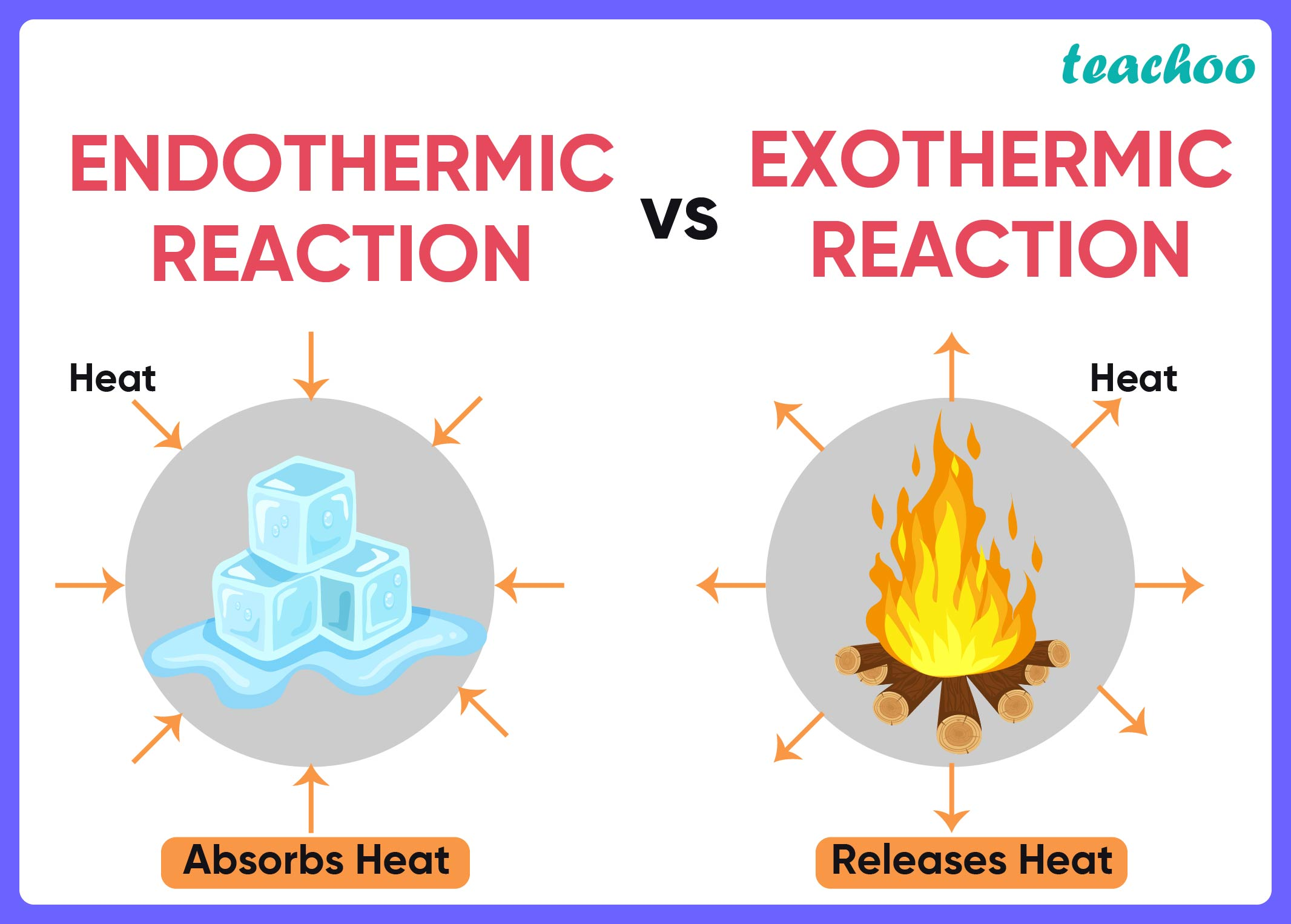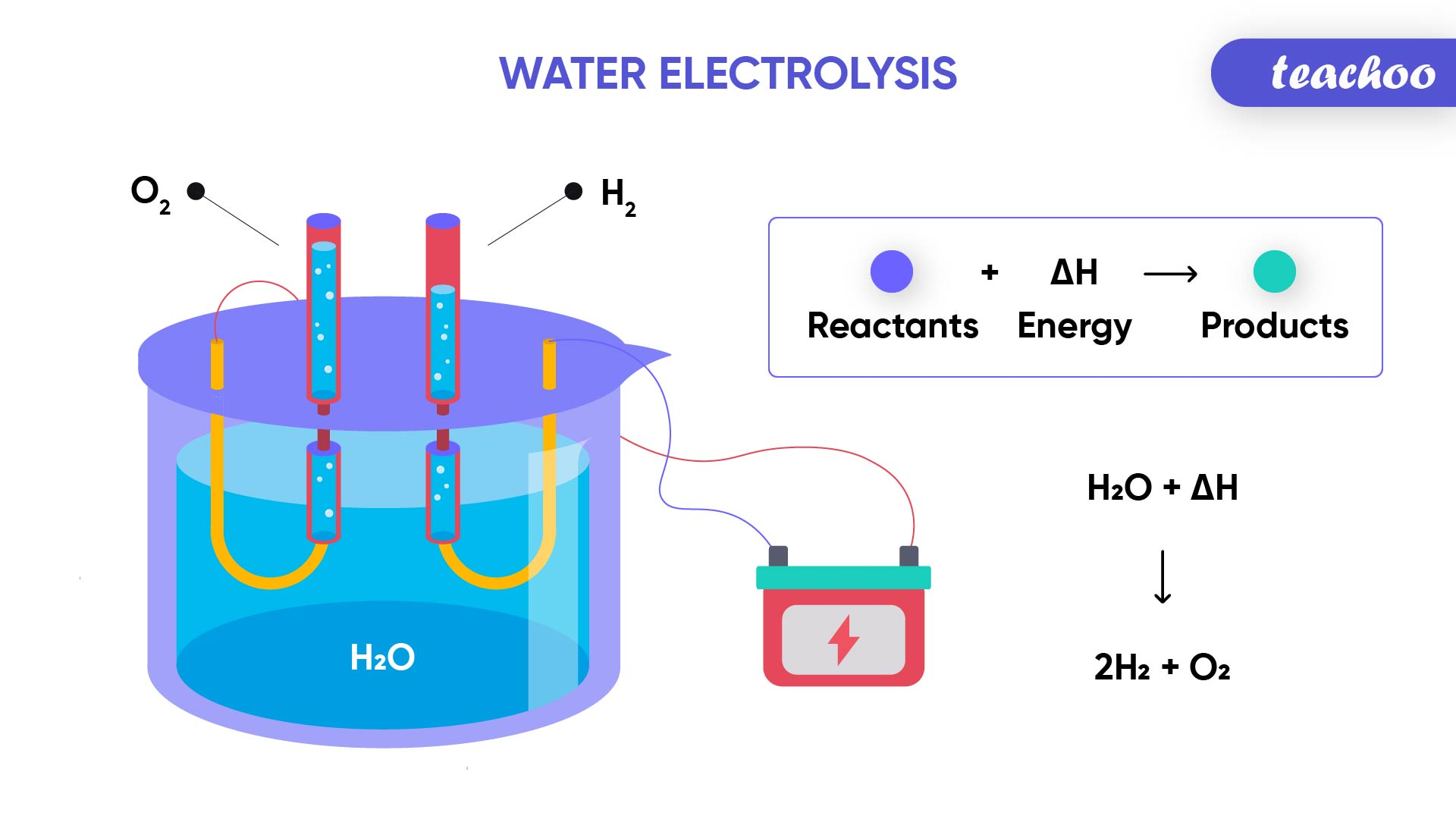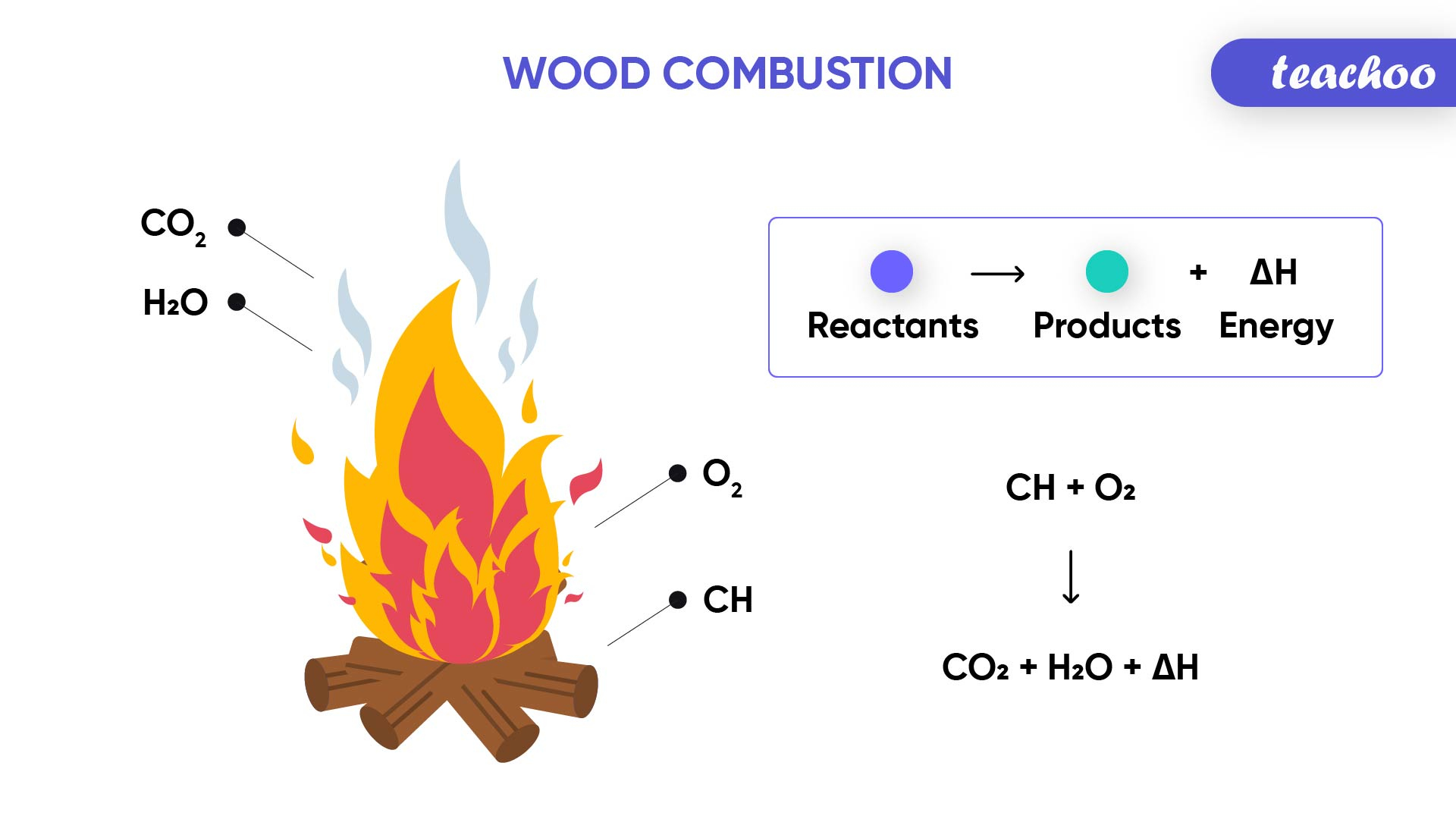Pressure-

For example

When Carbon Monoxide reacts with Hydrogen gas at a pressure of 250 atmospheres, Methanol is formed.

CO + 2H 2 →  CH 3 OH

Intext Question -

NCERT Exercise -

Introducing your new favourite teacher - Teachoo Black, at only ₹83 per month